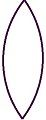• ×
الأربعاء 22 مايو 2019 | 05-12-2019

# Difference Between Convex and Concave LensThe lens is understood as a curved and transparent piece of glass or plastic, which focuses and refracts light rays in a certain manner. The curvature of the object ascertains the extent to which light is bent and in which direction. They are used in spectacles, microscope and telescopes. Based on the shape, lens can be grouped as a convex lens or concave lens. The former brings together the beam of light, while the latter disperses it. These two types of lenses are often understood together in physics and are commonly misinterpreted by the people. However, there are vast differences between convex and concave lens, which lies in their structure. So, take a look at the differences amidst these two.

BASIS FOR COMPARISONCONVEX LENSCONCAVE LENS
Meaning Convex lens refers to the lens which merges the light rays at a particular point, that travels through it. Concave lens can be identified as the lens which disperses the light rays around, that hits the lenses.
FigureCurve Outward Inward
Light Converges Diverges
Centre and edges Thicker at the center, as compared to its edges. Thinner at the center as compared to its edges.
Focal length Positive Negative
Image Real and inverted image. Virtual, erect and magnified image.
Objects Appear closer and larger. Appear smaller and farther.
Used to Correct hyperopia. Correct myopia.

### Definition of Convex Lens

Convex Lenses are the lenses that feel massive at the centre than at the edges. The curve of the lens is outward, and as the light beams pass through the lens, it refracts them and brings them together, resulting in the convergence of light, due to which it is also named as a converging lens. Look at the figure given below:So, the point where the light rays meet is known as a focal point, or principal focus and space amidst the centre of the lens and the principal focus is the focal length. Further, it generates a real and inverted image, but it can also form a virtual image when the object is placed too close to the lens. Such lenses are used to focus a beam of light on making the object look clearer and larger.

Example: The lenses of a camera are a convex lens, as the light rays focus on person or object being captured.

### Definition of Concave Lens

Concave lenses represent the type of lenses which are slender at the centre than at the borders. The shape of a concave lense is round inward that bends the beams outward, causing divergence of the rays of light falling on it, so it is known as a diverging lens. This also makes the object look smaller and farther than they really are and the image formed is virtual, diminished and upright.As you can see in the given figure, the light rays appear to be diverging from a virtual point, which is known as principal focus or focal point. Further, the length between the focal point and the centre of the lens is called focal length.

Example: Concave lenses are used in the side mirrors of cars and motorbikes. They can also be used in movie projectors to spread the image.

## Key Difference Convex and Concave Lens

The following points are noteworthy, so far as the difference between convex and concave lens is concerned:

1. The lens which merges the light rays at a particular point, that travels through it, are a convex lens. The lens which disperses the light rays around, that hits the lenses, are called a concave lens.
2. In the convex lens, the curve is outward facing, whereas, in the concave lens, the curve faces inward.
3. When the light rays pass through the convex lens, it converges the light rays and focuses on one point. On the other hand, when the light rays go through the concave lens, it diverges the beams, i.e. they spread out.
4. The structure of convex lens is like, thicker at the centre and thinner at the edges. Conversely, the concave lenses are thinner at the centre and thicker at its edges, in structure.
5. The focal length of a convex lens is positive, while that of a concave lens is negative.
6. Generally, a convex lens forms a real image, but it can also create a virtual image when the object is in the middle of the focus and optical centre. On the contrary, the image formed by the concave lens is erect, virtual and smaller, than the object.
7. Due to the thicker centre of convex lenses, the objects are seen larger and closer. Unlike, concave lens, whose thin centre causes the object to look farther and smaller.
8. A convex lens is used to treat hyperopia or farsightedness. In contrast, the concave lens proves helpful in the treatment of myopia or shortsightedness.

### Conclusion

So, with the above examples and figures, you might have got a clear understanding of the difference between the two types of lenses. Many times, convex and concave lenses are used along to produce sharper, clear and better images.

المصدر

بواسطة :
05-13-2017 12:00 صباحاً  0  0  2083
التعليقات ( 0 )